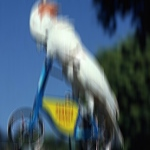# clojure2d.pixels

Operations on pixel levels.

## Content

Namespace defines three main concepts:

• Pixels - channel values packed into array.
• Processors - parallel Pixels processing functions (like filters).
• Bins - log density renderer

## Pixels

Pixels is type which represents image as int array divided into color channels. Layout is linear and interleaved which means that array is 1D and each pixel is represented by four consecutive values R, G, B, A. After first row goes second and so on.

Pixels allows mutation, you can read and set channel value or color:

Pixel access can be made by (x,y) coordinates or by index which is equivalent to (+ x (* y width)).

Pixels implement ImageProto.

### Creation / conversions

To create empty Pixels, call pixels.

You can also get and set Pixels from and to Images and Canvases or read from file.

## Processors

Library supports several processing functions and helpers to parallely manipulate channels or colors. All functions are not destrictive, that means new object is created to store result of manipulation. Every processor accept one or more filtering functions which do the job. There are three main functions:

• filter-colors - to process colors. Uses function f which accepts color and should return color. Can be used to convert Pixels between different color spaces.
• filter-channels - to process channel values. Uses function f which accepts channel number (values from 0 to 3), target Pixels and source Pixels and returns integer. You can provide different function for every channel. Can be used to apply filter (like blur).
• blend-channels - to process pair of Pixels. Uses function f which accepts channel number, target and two Pixel values. compose-channels wrapper can be used to compose two Pixels using one of the blending functions defined in clojure2d.colors namespace.

Additionally other processing functions are prepared in case you want write own filters or converters:

• filter-colors-xy - process colors using function f which accepts Pixels and current position.
• filter-channel - iterate through channel, f accepts channel value and returns new channel value
• filter-channel-xy - iterate through channel, f accepts channel, Pixels and x,y position
• blend-channels and blend-channel-xy - similar to two above, f accepts two Pixels instead of one.

### Color space

To convert whole Pixels into different color space use filter-colors and pass one of the color space conversion functions defined under colorspaces*. Always use normalized version.

(filter-colors c/to-HSB* pixels-object)


### Filters

There are several ready to use filters. All defined under filters-list variable. Some of the filters are creators and should be called with parametrization.

(filter-channels gaussian-blur-3 pixels-object)
(filter-channels (posterize 10) pixels-object)


### Composing

To compose two Pixels use compose-channels and use name of composing function defined blends-list. Instead of name you can pass custom composing function.

(compose-channels :multiply pixels-1 pixels-2)


## Log Density Rendering

Log Density Renderer was orginally created for fractal flames rendering and produces very smooth results. Details are described in this paper.

Renderer is point based (no other primitives) and supports selection of antialiasing (reconstruction) filters. Density estimation is not supported.

Rendering algorithm collects color channels values and counts number of hits for each pixel. For each pixel weighted average of all color values is calculated and log of number of hits gives alpha value. Pixel color is blended with background using alpha.

### Rendering

First you have to create renderer with renderer function. By default no filter is used. In case you want to use filter call with: filter name as keyword (see below), optional: filter radius (default 2.0) and other filter parameters.

To set point call set-color.

#### Antialiasing filters

Below you have list of all available antialiasing filters. Each filter has radius and spread parameter.

• :gaussian
• :sinc - Windowed sinc filter
• :mitchell - Mitchell-Netravali filter, additional parameters: B and C (default: 1/3)
• :cubic
• :catmull
• :triangle
• :cosinebell
• :blackmann-harris
• :hann
• :none or nil - no filter

### Converting

To convert renderer to Pixels just call to-pixels method with optional configuration. Configuration gives you possibility to control process of transformation to RGB data.

Configuration is a map with following fields:

• :background - color of the background (default: :black)
• :gamma-alpha - gamma correction for alpha
• :gamma-color - gamma correction for color, to adjust vibrancy
• :vibrancy: 0.0 - use calculated color 1.0 - use gamma corrected color (0.0-1.0) - mix between above
• :saturation - adjust saturation (0-2)
• :brightness - adjust brightness (0-2)
• :contrast - adjust contrast (0-2)

### Parallel rendering

Construction of renderer enables parallel computing. Just create as many renderers as you want (you may use available-tasks value), run rendering in separate threads and then merge result with merge-renderers.

#### Code snippets

Save pixels to image.

(defn saver
[f params & opts]
(let [n (str "images/pixels/" (first opts) ".jpg")]
(core/save (f) (str "docs/" n))
(str "../" n)))

### *pixels-edge*

#### dynamic

If you access pixels which is outside possible range. You’ll always get some value. You can control what is returned by setting this variable. Possible values are:

• :zero - set 0
• :edge - value from the edge of the image (left, right, top, bottom or corners) (default)
• :wrap - wrap around image
• or channel value 0-255 - set specific value

#### Examples

Access pixels outside image range.

(binding [*pixels-edge* :zero] (get-color cockatoo -1 10))
;;=> [0.0 0.0 0.0 255.0]
(get-color cockatoo 0 10)
;;=> [54.0 94.0 155.0 255.0]
(binding [*pixels-edge* :edge] (get-color cockatoo -1 10))
;;=> [54.0 94.0 155.0 255.0]
(get-color cockatoo (- (core/width cockatoo) 100) 10)
;;=> [186.0 102.0 76.0 255.0]
(binding [*pixels-edge* :wrap] (get-color cockatoo -100 10))
;;=> [186.0 102.0 76.0 255.0]
(binding [*pixels-edge* 123] (get-color cockatoo -1 10))
;;=> [123.0 123.0 123.0 255.0]

Get values from second channel from outside image range.

(binding [*pixels-edge* :zero] (get-value cockatoo 1 -1 10))
;;=> 0
(get-value cockatoo 1 0 10)
;;=> 94
(binding [*pixels-edge* :edge] (get-value cockatoo 1 -1 10))
;;=> 94
(get-value cockatoo 1 (- (core/width cockatoo) 100) 10)
;;=> 102
(binding [*pixels-edge* :wrap] (get-value cockatoo 1 -100 10))
;;=> 102
(binding [*pixels-edge* 123] (get-value cockatoo 1 -1 10))
;;=> 123

(add-pixel! r x y)(add-pixel! r x y c)

### blend-channel

(blend-channel f ch target p1 p2)(blend-channel f)

Blend one channel, write result into target.

Blending function should two channel values and return new channel value.

This should be considered as helper function for blend-channels.

#### Examples

Create own blending function and apply to second channel of image and its blurred version.

(saver (fn []
(let [target (clone-pixels cockatoo)
wrong-avg (fn [v1 v2] (/ (+ v1 v2) 4.0))]
(blend-channel wrong-avg
1
target
cockatoo
(filter-channels box-blur-5 cockatoo))
target))
...)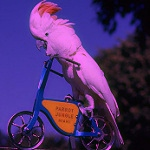### blend-channel-xy

(blend-channel-xy f ch target p1 p2)(blend-channel-xy f)

Blend one channel, write result into target.

Blending function should accept channel, two Pixels and position x,y.

#### Examples

Create flip blender and apply to second channel.

(saver (fn []
(let [target (clone-pixels cockatoo)
flip-blend (fn [ch p1 p2 x y]
(let [v1 (get-value p1 ch y x)
v2 (get-value p2 ch x y)]
(/ (+ v1 v2) 2.0)))]
(blend-channel-xy flip-blend 1 target cockatoo cockatoo)
target))
...)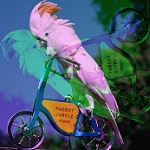### blend-channels

(blend-channels f0 f1 f2 f3 p1 p2)(blend-channels f p1 p2)(blend-channels f do-alpha? p1 p2)

Blend channels parallelly.

Similar to filter-channels. Bleding function should accept: channel, target and two source pixels.

#### Examples

Create flip blender and apply to image.

(saver (fn []
(let [flip-blend (fn [ch p1 p2 x y]
(let [v1 (get-value p1 ch y x)
v2 (get-value p2 ch x y)]
(/ (+ v1 v2) 2.0)))]
(blend-channels (partial blend-channel-xy flip-blend)
cockatoo
cockatoo)))
...)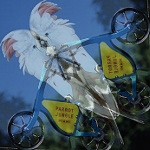### box-blur

(box-blur radius)

Create box blur for given radius.

#### Examples

Usage

(saver (fn [] (filter-channels (box-blur 20) cockatoo)) ...)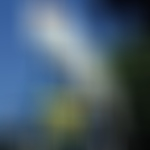### box-blur-1

#### Examples

Usage

(saver (fn [] (filter-channels box-blur-1 cockatoo)) ...)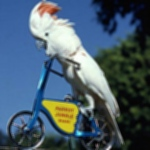### box-blur-2

#### Examples

Usage

(saver (fn [] (filter-channels box-blur-2 cockatoo)) ...)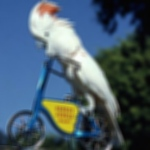### box-blur-3

#### Examples

Usage

(saver (fn [] (filter-channels box-blur-3 cockatoo)) ...)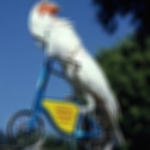### box-blur-5

#### Examples

Usage

(saver (fn [] (filter-channels box-blur-5 cockatoo)) ...)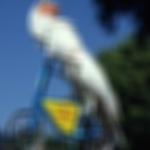### brightness-contrast

(brightness-contrast brightness contrast)(brightness-contrast brightness)

Create brightness / contrast filter.

Values from 0-2.

#### Examples

Ligher

(saver (fn [] (filter-channels (brightness-contrast 1.5) cockatoo)) ...)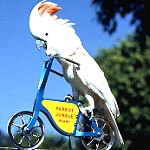Darker

(saver (fn [] (filter-channels (brightness-contrast 0.5) cockatoo)) ...)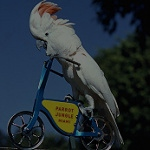More contrast

(saver (fn [] (filter-channels (brightness-contrast 1.0 1.5) cockatoo))
...)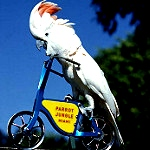Less contrast

(saver (fn [] (filter-channels (brightness-contrast 1.0 0.5) cockatoo))
...)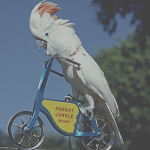### clone-pixels

(clone-pixels p)

Clone Pixels, returns new object

#### Examples

Usage

(let [p1 (pixels 100 100)]
(set-color! p1 10 10 :maroon)
(get-color (clone-pixels p1) 10 10))
;;=> [128.0 0.0 0.0 255.0]

### compose-channels

(compose-channels n1 n2 n3 n4 p1 p2)(compose-channels n1 n2 n3 p1 p2)(compose-channels n p1 p2)(compose-channels n do-alpha? p1 p2)

Compose channels with blending functions.

You can give blending method name as keyword defined in blends-names. Or it can be blending function which accepts 2 doubles from 0.0 to 1.0 and returns double (0.0 - 1.0).

When there is no processing function for alpha, W3C alpha blending is applied.

#### Examples

Add two versions of the image

(saver
(fn []
(compose-channels :add cockatoo (filter-channels solarize cockatoo)))
...)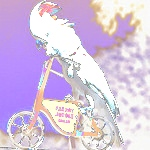XOR two versions of the image

(saver
(fn []
(compose-channels :xor cockatoo (filter-channels solarize cockatoo)))
...)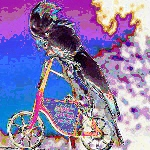Use different blending method for every channel.

(saver (fn []
(compose-channels :divide
:multiply
:mburn
cockatoo
(filter-channels solarize cockatoo)))
...)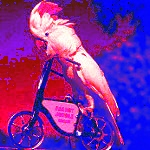Use custom function

(saver (fn []
(compose-channels (fn [v1 v2] (* v1 (- 1.0 v2)))
cockatoo
(filter-channels solarize cockatoo)))
...)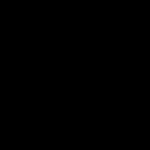### composite

(composite n)

Create java.awt.Composite object which can be used in set-composite.

Used to change default blending during drawing.

### dilate

Dilate filter. See: dilate-cross.

#### Examples

Apply filter 5 times.

(saver (fn []
(reduce (fn [buff _] (filter-channels dilate buff))
cockatoo
(range 5)))
...)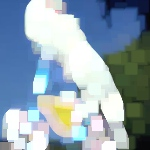### dilate-cross

Dilate using 5 pixels. See: dilate.

#### Examples

Apply filter 5 times.

(saver (fn []
(reduce (fn [buff _] (filter-channels dilate-cross buff))
cockatoo
(range 5)))
...)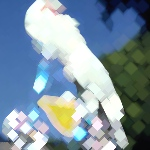### equalize

(equalize ch target p)

Equalize histogram.

#### Examples

Usage

(saver (fn [] (filter-channels equalize cockatoo)) ...)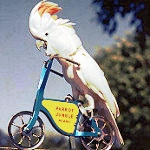### erode

Erode filter. See: erode-cross.

#### Examples

Apply filter 5 times.

(saver (fn []
(reduce (fn [buff _] (filter-channels erode buff))
cockatoo
(range 5)))
...)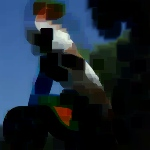### erode-cross

Erode using 5 pixels. See: erode.

#### Examples

Apply filter 5 times.

(saver (fn []
(reduce (fn [buff _] (filter-channels erode-cross buff))
cockatoo
(range 5)))
...)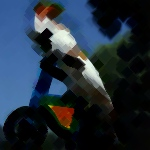### filter-channel

(filter-channel f ch target p)(filter-channel f)

Filter one channel, write result into target.

This is helper function to create own filters.

Function parameter is channel value and should return new value.

#### Examples

Operate on blue channel

(saver (fn []
(let [target (pixels 150 150)
filter (fn [v] (- 255 (/ v 2)))]
(filter-channel filter 2 target cockatoo)
target))
...)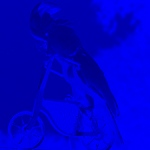### filter-channel-xy

(filter-channel-xy f ch target p)(filter-channel-xy f)

Filter one channel, write result into target.

This is helper function to create own filter.

Function parameters are: channel, pixels, x and y position.

Note: channel is first parameter.

#### Examples

Create filter and process channel.

(saver (fn []
(let [target (pixels 150 150)
filter (fn [ch p x y]
(let [v1 (get-value p ch (dec x) y)
v2 (get-value p ch (inc x) y)
v3 (get-value p ch x (dec y))
v4 (get-value p ch x (inc y))
avg (/ (+ v1 v2 v3 v4) 4.0)]
(if (< avg 128) 0 255)))]
(filter-channel-xy filter 0 target cockatoo)
(let [c0 (get-channel target 0)]
(set-channel! target 1 c0)
(set-channel! target 2 c0))
target))
...)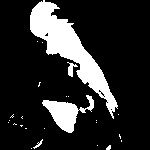Create filter and process channel. Using filter-channels.

(saver
(fn []
(let [filter (filter-channel-xy (fn [ch p x y]
(let [v1 (get-value p ch (dec x) y)
v2 (get-value p ch (inc x) y)
v3 (get-value p ch x (dec y))
v4 (get-value p ch x (inc y))
avg (/ (+ v1 v2 v3 v4) 4.0)]
(if (< avg 128) 0 255))))
target (filter-channels filter nil nil nil cockatoo)]
(let [c0 (get-channel target 0)]
(set-channel! target 1 c0)
(set-channel! target 2 c0))
target))
...)### filter-channels

(filter-channels f0 f1 f2 f3 p)(filter-channels f p)(filter-channels f do-alpha? p)

Filter channels parallelly with filtering function. Build filtering function using filter-channel or filter-channel-xy helpers as with filter aplied partially. Filtering function parameters are: channel, target and source pixels.

When you pass one filter, three RGB channels will be processed (arity: 2). To enable alpha set do-alpha parameter to true (arity: 3). You can also use different filter for every channel separately (arity 5). Set nil to skip particular channel.

#### Examples

Apply equalize filter for all channels

(saver (fn [] (filter-channels equalize cockatoo)) ...)Apply 2 filters separately for 2 channels

(saver (fn [] (filter-channels negate (box-blur 10) nil nil cockatoo))
...)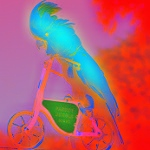Create gamma filter and use it to process channels

(saver
(fn []
(let [gamma-fn
(fn [amt v]
(let [vv (/ v 255.0) p (m/pow vv (/ amt))] (* p 255.0)))
my-gamma (partial gamma-fn 0.2)]
(filter-channels (partial filter-channel my-gamma) cockatoo)))
...)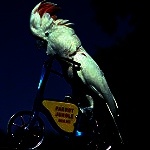### filter-colors

(filter-colors f p)

Filter colors.

Filtering function should accept color and return color.

#### Examples

Convert to other colorspace.

(saver (fn [] (filter-colors c/to-LUV* cockatoo)) ...)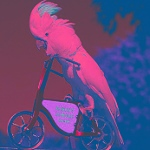### filter-colors-xy

(filter-colors-xy f p)

Filter colors.

Filtering function should accept Pixels, position as x,y values and return color

#### Examples

Shift image up.

(saver (fn []
(let [filter (fn [p x y] (get-color p x (+ y 75)))]
(binding [*pixels-edge* :wrap]
(filter-colors-xy filter cockatoo))))
...)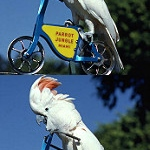### gaussian-blur

(gaussian-blur radius)

Create gaussian blur for given radius.

#### Examples

Usage

(saver (fn [] (filter-channels (gaussian-blur 20) cockatoo)) ...)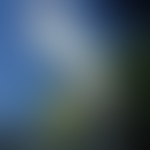### gaussian-blur-1

#### Examples

Usage

(saver (fn [] (filter-channels gaussian-blur-1 cockatoo)) ...)### gaussian-blur-2

#### Examples

Usage

(saver (fn [] (filter-channels gaussian-blur-2 cockatoo)) ...)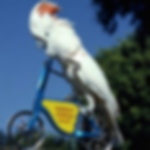### gaussian-blur-3

#### Examples

Usage

(saver (fn [] (filter-channels gaussian-blur-3 cockatoo)) ...)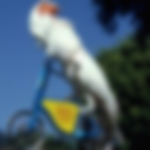### gaussian-blur-5

#### Examples

Usage

(saver (fn [] (filter-channels gaussian-blur-5 cockatoo)) ...)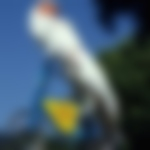### get-channel

(get-channel pixels ch)

Return whole ints array with chosen channel

#### Examples

Extract one channel from Pixels.

(first (get-channel cockatoo 1))
;;=> 91

### get-color

(get-color pixels x y)(get-color pixels idx)

Get color by index or position. In case of low density rendering returns current average color without alpha value.

#### Examples

Get channel value from given position.

(get-color cockatoo 20 20)
;;=> [60.0 100.0 161.0 255.0]
(get-color cockatoo -10 -10)
;;=> [51.0 91.0 152.0 255.0]
(get-color cockatoo 20)
;;=> [52.0 92.0 153.0 255.0]
(get-color cockatoo 10000)
;;=> [56.0 66.0 39.0 255.0]
(get-color (core/canvas 100 100) 50 50)
;;=> [0.0 0.0 0.0 0.0]
;;=> [176.0 191.0 184.0 255.0]

Low density pixels color access.

(let [ldp (renderer 100 100)
v0 (get-color ldp 50 50)
v1 (get-color (set-color! ldp 50 50 :maroon) 50 50)]
{:before v0, :after v1})
;;=> {:after [128.0 0.0 0.0 255.0],
;;=>  :before [##NaN ##NaN ##NaN 255.0]}

### get-pixel

(get-pixel r x y)

Get pixel (color) from renderer buffer

### get-value

(get-value pixels ch x y)(get-value pixels ch idx)

Get channel value by index or position.

#### Examples

Get channel value from given position.

(get-value cockatoo 1 20 20)
;;=> 100
(get-value cockatoo 3 20 20)
;;=> 255
(get-value cockatoo 0 -10 -10)
;;=> 51
(get-value cockatoo 1 20)
;;=> 92
(get-value cockatoo 3 20)
;;=> 255
(get-value cockatoo 0 10000)
;;=> 56

(gradient-renderer w h filter filter-radius & filter-params)(gradient-renderer w h filter)(gradient-renderer w h)

Optionally you can pass antialiasing filter and its parameters. Defaults to :none.

#### Examples

Usage

(saver
(fn* []
(dotimes [i 4000000]
(let [x (+ 150 (r/grand 30))
y (+ 150 (r/grand 30))
x (+ x
(* 20.0
(- (local-noise (/ x 50.0) (/ y 50.0) 0.34) 0.5)))
y (+ y
(* 20.0
(- (local-noise (/ y 50.0) (/ x 50.0) 2.23) 0.5)))]
(to-pixels r)))
...)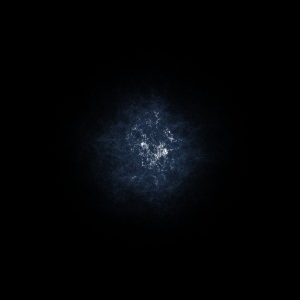(saver
(fn* []
(dotimes [i 4000000]
(let [x (+ 150 (r/grand 30))
y (+ 150 (r/grand 30))
x (+ x
(* 20.0
(- (local-noise (/ x 50.0) (/ y 50.0) 0.34) 0.5)))
y (+ y
(* 20.0
(- (local-noise (/ y 50.0) (/ x 50.0) 2.23) 0.5)))]
...)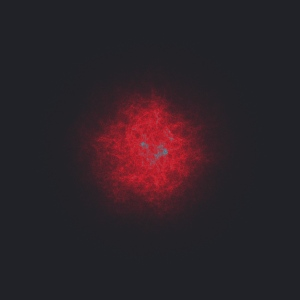Render with logarithmic scale

(saver
(fn* []
(dotimes [i 4000000]
(let [x (+ 150 (r/grand 30))
y (+ 150 (r/grand 30))
x (+ x
(* 20.0
(- (local-noise (/ x 50.0) (/ y 50.0) 0.34) 0.5)))
y (+ y
(* 20.0
(- (local-noise (/ y 50.0) (/ x 50.0) 2.23) 0.5)))]
(to-pixels r {:logarithmic? true})))
...)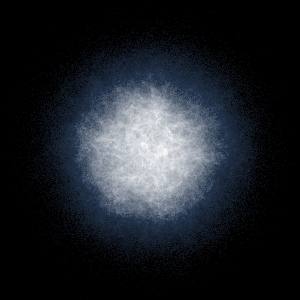### horizontal-blur

(horizontal-blur radius)

Create horizontal blur for given radius.

#### Examples

Usage

(saver (fn [] (filter-channels (horizontal-blur 20) cockatoo)) ...)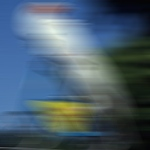### horizontal-blur-1

#### Examples

Usage

(saver (fn [] (filter-channels horizontal-blur-1 cockatoo)) ...)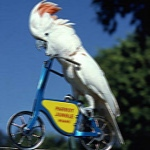### horizontal-blur-2

#### Examples

Usage

(saver (fn [] (filter-channels horizontal-blur-2 cockatoo)) ...)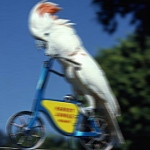### horizontal-blur-3

#### Examples

Usage

(saver (fn [] (filter-channels horizontal-blur-3 cockatoo)) ...)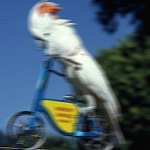### horizontal-blur-5

#### Examples

Usage

(saver (fn [] (filter-channels horizontal-blur-5 cockatoo)) ...)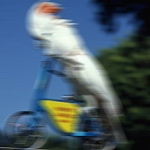(load-pixels n)

Load Pixels from file.

#### Examples

(load-pixels "docs/cockatoo.jpg")
;;=> pixels (150, 150)

Usage

(saver (fn [] (load-pixels "docs/cockatoo.jpg")) ...)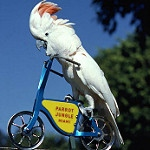### median

Median filter

#### Examples

Apply filter 5 times.

(saver (fn []
(reduce (fn [buff _] (filter-channels median buff))
cockatoo
(range 5)))
...)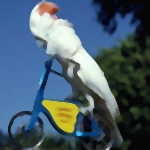(merge-gradient-renderers target & renderers)

Merge gradient renderers and store result to the target.

Use this function to merge separate rendereing results (ex. from separeted threads).

This is mutating function. Data from list of renderers is added to the target.

### merge-renderers

(merge-renderers target & renderers)

Paralelly merge renderers and store result to the target.

Use this function to merge separate rendereing results (ex. from separeted threads).

This is mutating function. Data from list of renderers is added to the target.

### modulate

(modulate amt)

Create modulate channel values filter.

Great to work with hue based color spaces.

Values from 0-2.

#### Examples

Saturate

(saver (fn []
(->> cockatoo
(filter-colors c/to-HSB*)
(filter-channels nil (modulate 2.0) nil nil)
(filter-colors c/from-HSB*)))
...)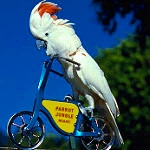Desaturate

(saver (fn []
(->> cockatoo
(filter-colors c/to-HCL*)
(filter-channels nil (modulate 0.2) nil nil)
(filter-colors c/from-HCL*)))
...)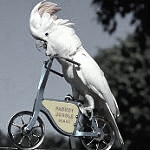Shift hue

(saver (fn []
(->> cockatoo
(filter-colors c/to-HCL*)
(filter-channels (modulate 0.2) nil nil nil)
(filter-colors c/from-HCL*)))
...)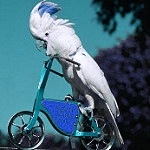### negate

(negate ch target p)

Negate filer.

### normalize

(normalize ch target p)

Normalize channel values to full range.

#### Examples

Usage

(saver (fn [] (filter-channels normalize cockatoo)) ...)### pixels

(pixels a w h)(pixels w h)

Create empty Pixels object with w,h dimensions.

Optionally you can pass ints array a as a buffer. Size of array should be (* 4 w h).

#### Examples

Create pixels

(pixels 100 100)
;;=> pixels (100, 100)

Create pixels with given array

(pixels (int-array (* 10 10 4)) 10 10)
;;=> pixels (10, 10)

### posterize

(posterize levels)

Create posterize filter for given radius.

#### Examples

Usage

(saver (fn [] (filter-channels (posterize 2) cockatoo)) ...)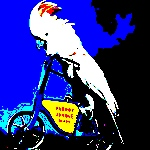### posterize-16

#### Examples

Usage

(saver (fn [] (filter-channels posterize-16 cockatoo)) ...)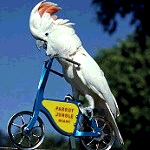### posterize-4

#### Examples

Usage

(saver (fn [] (filter-channels posterize-4 cockatoo)) ...)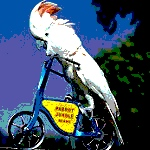### posterize-8

#### Examples

Usage

(saver (fn [] (filter-channels posterize-8 cockatoo)) ...)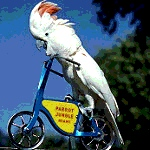### quantile-1

Quantile (2/9) filter

#### Examples

Apply filter 5 times.

(saver (fn []
(reduce (fn [buff _] (filter-channels quantile-1 buff))
cockatoo
(range 5)))
...)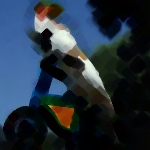### quantile-2

Quantile (3/9) filter

#### Examples

Apply filter 5 times.

(saver (fn []
(reduce (fn [buff _] (filter-channels quantile-2 buff))
cockatoo
(range 5)))
...)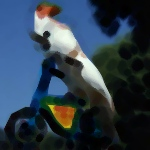### quantile-3

Quantile (4/9) filter

#### Examples

Apply filter 5 times.

(saver (fn []
(reduce (fn [buff _] (filter-channels quantile-3 buff))
cockatoo
(range 5)))
...)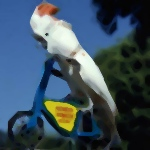### quantile-5

Quantile (6/9) filter

#### Examples

Apply filter 5 times.

(saver (fn []
(reduce (fn [buff _] (filter-channels quantile-5 buff))
cockatoo
(range 5)))
...)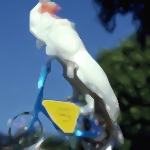### quantile-6

Quantile (7/9) filter

#### Examples

Apply filter 5 times.

(saver (fn []
(reduce (fn [buff _] (filter-channels quantile-6 buff))
cockatoo
(range 5)))
...)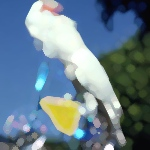### quantile-7

Quantile (8/9) filter

#### Examples

Apply filter 5 times.

(saver (fn []
(reduce (fn [buff _] (filter-channels quantile-7 buff))
cockatoo
(range 5)))
...)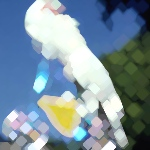### renderer

(renderer w h filter filter-radius & filter-params)(renderer w h filter)(renderer w h)

Create renderer.

Optionally you can pass antialiasing filter and its parameters. Defaults to :none.

#### Examples

Log density rendering

(saver
(fn* []
(let [r (renderer 300 300)]
(dotimes [i 20000000]
(let [x (+ 150 (r/grand 30))
y (+ 150 (r/grand 30))
x (+ x
(* 20.0
(- (local-noise (/ x 50.0) (/ y 50.0) 0.34) 0.5)))
y (+ y
(* 20.0
(- (local-noise (/ y 50.0) (/ x 50.0) 2.23) 0.5)))]
(to-pixels r {:gamma-alpha 0.6, :gamma-color 0.8})))
...)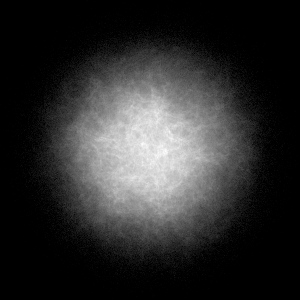Linear density rendering

(saver
(fn* []
(let [r (renderer 300 300)]
(dotimes [i 20000000]
(let [x (+ 150 (r/grand 30))
y (+ 150 (r/grand 30))
x (+ x
(* 20.0
(- (local-noise (/ x 50.0) (/ y 50.0) 0.34) 0.5)))
y (+ y
(* 20.0
(- (local-noise (/ y 50.0) (/ x 50.0) 2.23) 0.5)))]
(to-pixels r {:gamma-alpha 0.6, :gamma-color 0.8, :linear? true})))
...)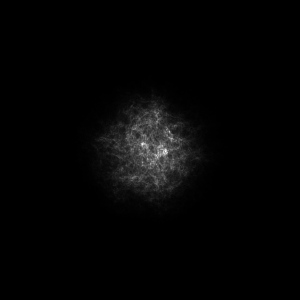Splat rendering

(saver
(fn* []
(let [r (renderer 300 300 :gaussian)]
(doseq [x (range 300)
y (range 300)]
(dotimes [i 10]
(let [xx (+ x (r/drand))
yy (+ y (r/drand))]
r
x
y
(c/gray (* 255.0 (local-noise (/ xx 50.0) (/ yy 50.0))))))))
(to-pixels r {:gamma-color 0.8, :splats? true})))
...)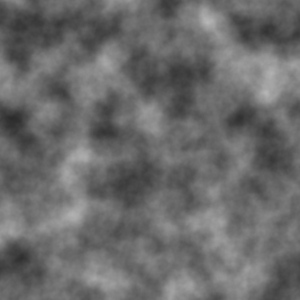Compare to native Java2d rendering. You can observe oversaturation.

(saver
(fn* []
(let [r (core/black-canvas 300 300)]
(core/with-canvas
[c r]
(core/set-color c :white 5)
(dotimes [i 2000000]
(let [x (+ 150 (r/grand 30))
y (+ 150 (r/grand 30))
x (+ x
(* 20.0
(- (local-noise (/ x 50.0) (/ y 50.0) 0.34) 0.5)))
y (+ y
(* 20.0
(- (local-noise (/ y 50.0) (/ x 50.0) 2.23)
0.5)))]
(core/point c x y))))
(to-pixels r)))
...)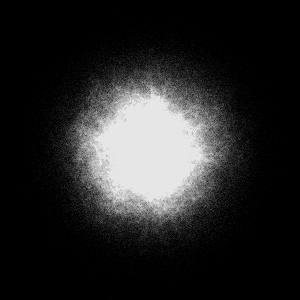### set-canvas-pixels!

(set-canvas-pixels! canvas x y p)(set-canvas-pixels! canvas p)

Set Pixels to canvas. See set-image-pixels!.

#### Examples

Usage

(saver
(fn []
(let [i (core/canvas 100 100)
p2 (pixels 40 40)]
(core/with-canvas [c i]
(core/set-background c :maroon)
(to-pixels (set-canvas-pixels! c 25 15 p2)))))
...)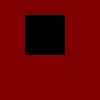### set-channel!

(set-channel! pixels ch v)

Set whole channel (as ints array)

#### Examples

Copy channels

(get-color
(set-channel! (clone-pixels cockatoo) 0 (get-channel cockatoo 1))
20
20)
;;=> [100.0 100.0 161.0 255.0]

### set-color!

(set-color! pixels x y v)(set-color! pixels idx v)

Set color value by index or position

#### Examples

Set color in pixels

(let [p (pixels 100 100)
v0 (get-color p 50 50)
v1 (get-color (set-color! p 50 50 :maroon) 50 50)]
{:before v0, :after v1})
;;=> {:after [128.0 0.0 0.0 255.0], :before [0.0 0.0 0.0 0.0]}

Low density pixels color access.

(let [ldp (renderer 100 100)
v0 (get-color ldp 50 50)
v1 (get-color (set-color! ldp 50 50 :maroon) 50 50)]
{:before v0, :after v1})
;;=> {:after [128.0 0.0 0.0 255.0],
;;=>  :before [##NaN ##NaN ##NaN 255.0]}

### set-image-pixels!

(set-image-pixels! b x y pin)(set-image-pixels! b p)

Set Pixels to image, mutating it.

Optionally you can set position when Pixels object has smaller size.

#### Examples

Usage

(saver (fn []
p2 (pixels 40 40)]
(to-pixels (set-image-pixels! i 25 15 p2))))
...)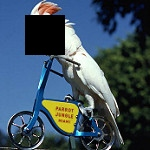### set-renderer-scaling-factor!

(set-renderer-scaling-factor! renderer ch1-scale ch2-scale ch3-scale)(set-renderer-scaling-factor! renderer scale)

Changes internal representation of density renderer values.

By default each channel is divided by 255.0 (and later multiplied by 255.0). Other values can be used to scale down and up other than RGB color ranges.

The main goal is to store values from 0.0 to 1.0.

### set-value!

(set-value! pixels ch x y v)(set-value! pixels ch idx v)

Set channel value by index or position

#### Examples

Set value in pixels

(let [p (pixels 100 100)
v0 (get-value p 1 50 50)
v1 (get-value (set-value! p 50 50 111) 50 50)]
{:before v0, :after v1})
;;=> {:after 111, :before 0}

### solarize

(solarize ch target p)

Solarize filter.

#### Examples

Usage

(saver (fn [] (filter-channels solarize cockatoo)) ...)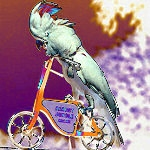### threshold

(threshold amount)(threshold amount-low amount-high)

Create threshold filter.

You can pass amount from 0-1. or range.

Note if you want b&w result first convert to gray color.

#### Examples

Usage

(saver (fn [] (filter-channels (threshold 0.9) cockatoo)) ...)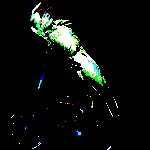Usage (two parameters)

(saver (fn [] (filter-channels (threshold 0.6 0.9) cockatoo)) ...)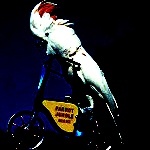Usage on b&w

(saver (fn []
(filter-channels (threshold 0.4)
(filter-colors c/to-Gray* cockatoo)))
...)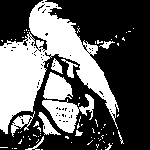### threshold-25

#### Examples

Usage

(saver (fn [] (filter-channels threshold-25 cockatoo)) ...)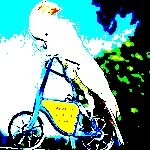### threshold-50

#### Examples

Usage

(saver (fn [] (filter-channels threshold-50 cockatoo)) ...)### threshold-75

#### Examples

Usage

(saver (fn [] (filter-channels threshold-75 cockatoo)) ...)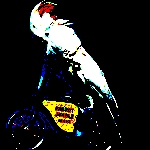### tint

(tint col)(tint col-low col-high)(tint col-low col-mid col-high)

Create tinting filter.

• one color - tint
• two colors - interpolate between col-low for black and col-high for white.
• three colors - like above but uses also mid-color for mid tones.

#### Examples

Usage

(saver (fn [] (filter-channels (tint :hotpink) cockatoo)) ...)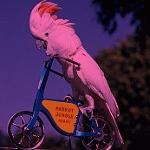Usage

(saver (fn [] (filter-channels (tint :indigo :lightblue) cockatoo)) ...)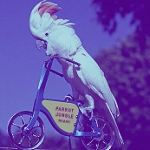Usage

(saver (fn []
(filter-channels (tint :gray :lightblue :maroon) cockatoo))
...)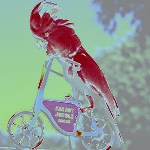### to-pixels

(to-pixels pixels)(to-pixels pixels x y w h)(to-pixels pixels cfg)

Convert to Pixels. For low density rendering provide configuration. Works with Image/Canvas/Window and low density renderer.

#### Examples

Convert various types to pixels

(to-pixels (pixels 100 100))
;;=> pixels (100, 100)
;;=> pixels (150, 150)
(to-pixels (core/canvas 50 50))
;;=> pixels (50, 50)
(to-pixels (renderer 10 10))
;;=> pixels (10, 10)

### vertical-blur

(vertical-blur radius)

Create vertical blur for given radius.

#### Examples

Usage

(saver (fn [] (filter-channels (vertical-blur 20) cockatoo)) ...)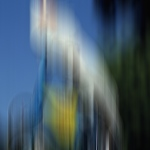### vertical-blur-1

#### Examples

Usage

(saver (fn [] (filter-channels vertical-blur-1 cockatoo)) ...)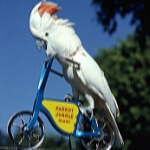### vertical-blur-2

#### Examples

Usage

(saver (fn [] (filter-channels vertical-blur-2 cockatoo)) ...)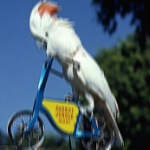### vertical-blur-3

#### Examples

Usage

(saver (fn [] (filter-channels vertical-blur-3 cockatoo)) ...)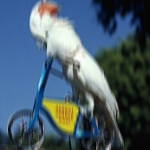### vertical-blur-5

#### Examples

Usage

(saver (fn [] (filter-channels vertical-blur-5 cockatoo)) ...)